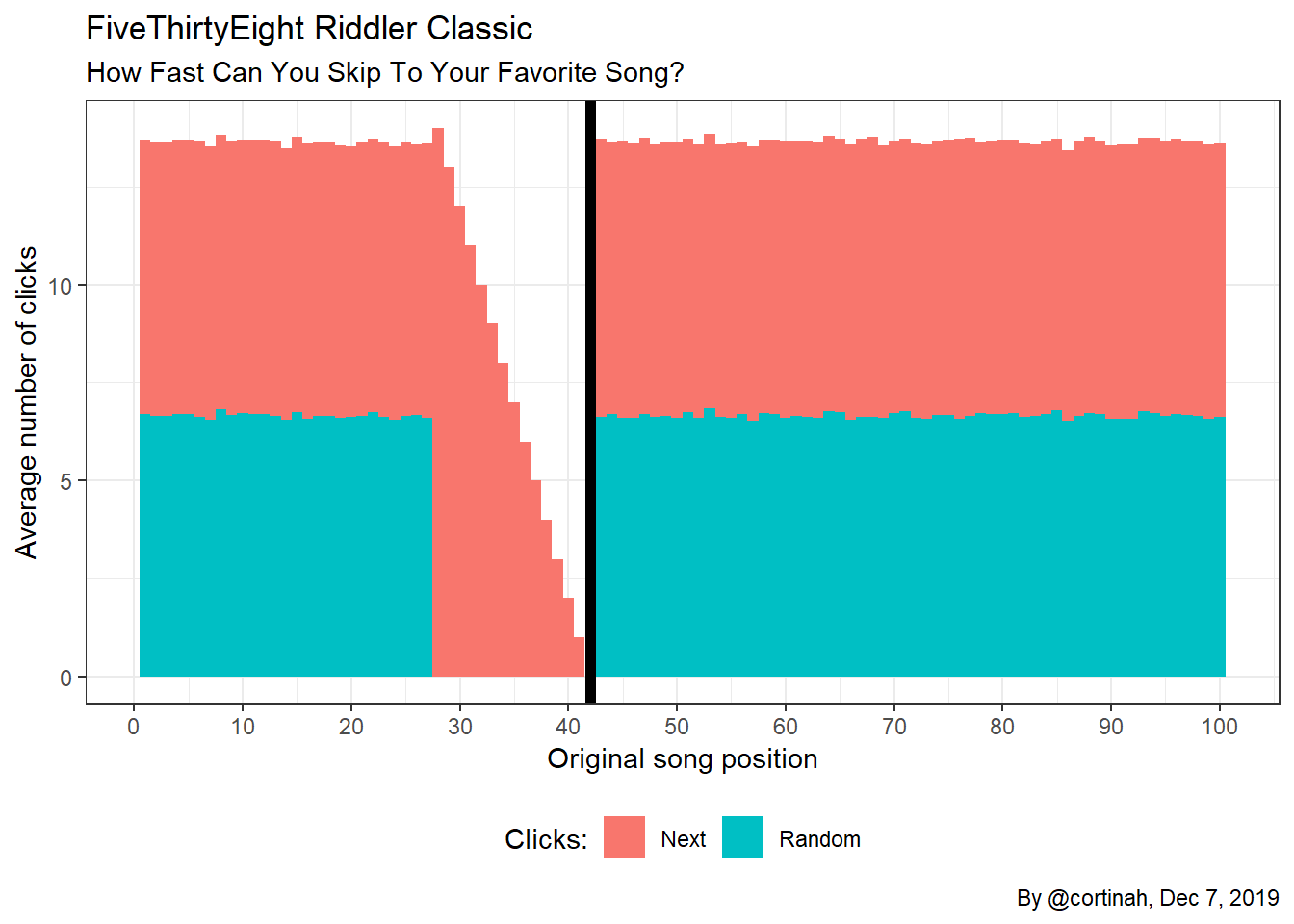In this week’s FiveThirtyEight Riddler Classic we are asked for the optimal strategy to play song number 42 on your 100-song playlist, starting from a random position, with only “next song”, and “random song” buttons. Also, on average how many clicks will you need to get to that song?

## Proposed Solution

We set r between 0 and 0.5 as the breakpoint above which we use the “next” button. We always use the random key if the current position is between 43 and 100.

$\text{Number of key presses} = random + next = \frac{1}{0.42-r} + \frac{0.42-r}{2}\dot{}100$ The number of “random” clicks is the reciprocal of the probability of falling in the range. The number of “next” key presses is (0.42 - r)*100, which needs to be divided by two because the expected value would be in the middle of the range.

Setting the first derivative to zero to minimize, we have:

$\frac{1}{(0.42-r)^{2}}-50 =0$

Which has a root at 0.2785, which we round to 0.28, or song 28. Accordingly, we should keep pressing the “random” key unless the current song is between numbers 28 and 42.

Let’s simulate this strategy with one million trials, and look at the distribution of results and the average number of clicks needed by following this strategy.

library(tidyverse)

playlist <- function(rounds=100){

results <- matrix(NA, ncol=3, nrow = rounds)
colnames(results) <- c('origin', 'arrows', 'randoms')

for (i in seq_len(rounds)) {
original_position <- sample.int(n = 100, size = 1)
random_clicks <- arrow_clicks <- 0
results[i, 1] <- original_position

while ((original_position > 42) | (original_position < 28))  {

random_clicks <- random_clicks + 1
original_position <- sample.int(n = 100, size = 1)
}
arrow_clicks <- (42 - original_position)
results[i, 2:3] <- c(arrow_clicks, random_clicks)
}
return(as.data.frame(results))
}

plays <- playlist(1000000)

plays_sum <- plays %>% group_by(origin) %>%
summarize(arrows=mean(arrows), randoms=mean(randoms))

plays %>% summarize(clicks=round(mean(arrows+randoms),1))
##   clicks
## 1   12.7

## Plotting the ResultsThe chart shows the number of clicks of the “next” and “random” keys from every starting position. When you start below, but within 14 songs of 42, the “random” key isn’t used. It takes on average about 12.7 total key presses to get to song 42! If you had both a “next” and “previous” key, it would only take 9 key pressses on average.